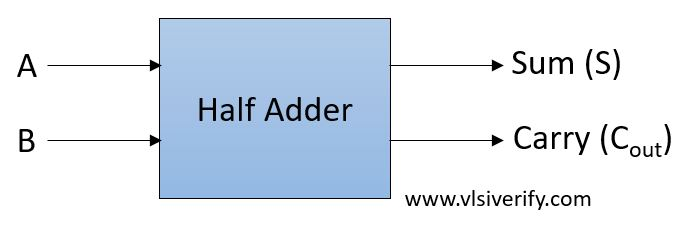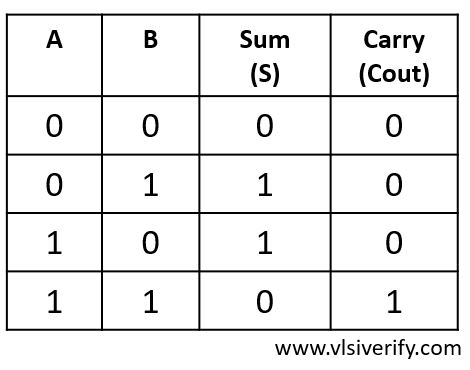Tutorials

Half Adder is a basic combinational design that can add two single bits and results to a sum and carry bit as an output.

Block DiagramTruth TableOutput:
S = A ^ B

Cout = A · B

As half adder considers only two bits so along with the addition of two single bits, it can not accommodate an extra carry bit from the previously generated result. Hence, it is called a half-adder. A full adder is designed to accommodate the extra carry bit from the previous stage.

``````module half_adder(input a, b, output s, Cout);
assign S = a ^ b;
assign Cout = a & b;
endmodule``````

### Testbench Code

``````module tb_top;
reg a, b;
wire s, c_out;

initial begin
\$monitor("At time %0t: a=%b b=%b, sum=%b, carry=%b",\$time, a,b,s,c_out);
a = 0; b = 0;
#1;
a = 0; b = 1;
#1;
a = 1; b = 0;
#1;
a = 1; b = 1;
end
endmodule``````

Output:

``````At time 0: a=0 b=0, sum=z, carry=0
At time 1: a=0 b=1, sum=z, carry=0
At time 2: a=1 b=0, sum=z, carry=0
At time 3: a=1 b=1, sum=z, carry=1``````

Verilog Codes